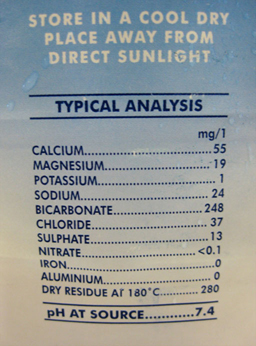Science, Maths & Technology

### Become an OU studentDiscovering chemistry

Start this free course now. Just create an account and sign in. Enrol and complete the course for a free statement of participation or digital badge if available.

# 3.3 Working with solutions: concentration

In making a solution, the chemicals involved are dissolved in a liquid which is referred to as the solvent.

The substance that dissolves is called the solute.

The most common solvent is water, but in the laboratory a chemist may choose to work with any one of a number of others. Different substances dissolve more easily or less easily in a particular solvent i.e. they have different solubilities. Here the solubility of a substance is defined as the maximum amount of solute that will dissolve in a given amount of solvent.

For example, when common salt (sodium chloride, the solute) is dissolved in water (the solvent) this gives a solution of sodium chloride. The solubility of a substance varies with temperature and is usually expressed as the maximum amount of a substance that will dissolve in 100 g of water at a particular temperature. However, it is often more usual (and more useful) to express the amount of solute that is dissolved in a specific volume of solution at a particular temperature.

This is known as the concentration of a solution, which is expressed as the amount of solute in 1dm 3.

Thus, if 10.0 g of sodium chloride is dissolved in water to give 1 dm3 of solution, the concentration would be 10.0 g per dm3 and so we would write 10.0 g dm3.

One important point: it is essential to define concentration as the amount of solute in exactly 1 dm 3, that is in 1 dm 3 of the final solution rather than the amount of solute dissolved in 1 dm3 of water. If you did dissolve 10.0 g of sodium chloride in 1 dm3 of water then the final volume would in effect be greater than 1 dm3. Therefore to make up a solution with a particular concentration of a solute, the required amount of solute is firstly dissolved fully in a suitable amount of water (less than 1 dm3) and then sufficient water is added to achieve the correct final volume of 1 dm3 .

Note 1dm3 = 1 litre

This is a label from a bottle of mineral water. It shows the concentration of each of the solutes expressed as mg/l.Figure 8 Label from a bottle of mineral water